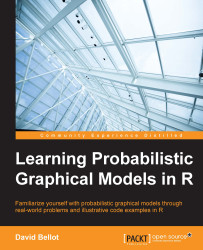•#### Learning Probabilistic Graphical Models in R#### Overview of this book

Probabilistic graphical models (PGM, also known as graphical models) are a marriage between probability theory and graph theory. Generally, PGMs use a graph-based representation. Two branches of graphical representations of distributions are commonly used, namely Bayesian networks and Markov networks. R has many packages to implement graphical models. We’ll start by showing you how to transform a classical statistical model into a modern PGM and then look at how to do exact inference in graphical models. Proceeding, we’ll introduce you to many modern R packages that will help you to perform inference on the models. We will then run a Bayesian linear regression and you’ll see the advantage of going probabilistic when you want to do prediction. Next, you’ll master using R packages and implementing its techniques. Finally, you’ll be presented with machine learning applications that have a direct impact in many fields. Here, we’ll cover clustering and the discovery of hidden information in big data, as well as two important methods, PCA and ICA, to reduce the size of big problems.
Learning Probabilistic Graphical Models in RCreditswww.PacktPub.comPrefaceFree Chapter
Probabilistic ReasoningExact InferenceLearning ParametersBayesian Modeling – Basic ModelsApproximate InferenceBayesian Modeling – Linear ModelsProbabilistic Mixture ModelsAppendixIndex## Summary

In this first chapter we learned the base concepts of probabilities

We saw how and why they are used to represent uncertainty about data and knowledge, while also introducing the Bayes formula. This is the most important formula to compute posterior probabilities—that is, to update our beliefs and knowledge about a fact when new data is available

We saw what a joint probability distribution is and learnt that they can quickly become too complex and intractable to deal with. We learned the basics of probabilistic graphical models as a generic framework to perform tractable, efficient, and easy modeling with probabilistic models. Finally, we introduced the different types of probabilistic graphical model and learned how to use R packages to write our first models

In the next chapter, we will learn the first set of algorithms to do Bayesian inference with probabilistic graphical models—that is, to put questions and queries to our models. We will introduce new features of the R packages and, at the same time, we'll learn how these algorithms work and can be used in an efficient manner.